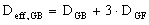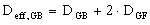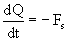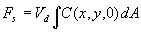c. Two-Month Meteorological Reanalysis

During the Gulf War, several other incidents occurred that possibly released chemical warfare agents. Investigating these possible releases’ effects also would require the prevailing meteorological conditions. Therefore, DHS asked the three meteorological modeling groups (NCAR for the MM5 model, DTRA for the OMEGA model, and NRL for the COAMPS model) to perform a reanalysis, using the same configuration found best for the Khamisiyah analysis, for the entire Persian Gulf War period, i.e., the two months from January 16 through March 15, 1991. This two-month reanalysis created a valuable database (more than 400GB in size) of predicted meteorological fields over the region. If investigators need to study a different incident, it is only a matter of retrieving the meteorological data for the corresponding geographical area and dates and then conducting dispersion modeling.

Since the two dispersion models (HPAC/SCIPUFF and VLSTRACK) cannot directly use the outputs from the three mesoscale meteorological models (COAMPS, MM5, and OMEGA), additional processing or reformatting is necessary. Previously, the two dispersion modeling groups did the processing separately as needed. As a result, the process was somewhat tedious and had some minor inconsistencies. Moreover, the MM5 and OMEGA fields were not used to run VLSTRACK in 1997 because the necessary processing software could not be developed in time. As part of the two-month reanalysis effort, conversion programs were developed to efficiently and consistently extract and process the meteorological model outputs so the dispersion models can use them directly (Sarma et al., 2000).

To be consistent with the 1997 results, the 2000 Khamisiyah analysis still includes the same four model combinations:

 •COAMPSHPAC/SCIPUFF •OMEGAHPACSCIPUFF •MM5HPAC/SCIPUFF •COAMPSVLSTRACK

Including the OMEGA/VLSTRACK and MM5/VLSTRACK combinations does not change the final exposure estimates based on the ensemble (or union) approach, because the hazard areas VLSTRACK predicts almost always are enclosed by those HPAC/SCIPUFF predicts. This is because VLSTRACK calculates median (50%) concentrations and HPAC/SCIPUFF’s results account for concentration fluctuations (with a 1% probability of exceeding the nominal value).

d. Performance Evaluation for Two-Month Meteorological Reanalysis

The performance of the three mesoscale meteorological models for the two-month reanalysis was evaluated by comparing the model results with rawinsonde and surface observations available in the area. Performance evaluation included temperature, dew point, wind speed, and wind direction. The same performance statistics were considered, including the mean error (ME), the mean absolute error (MAE), and the root mean square error (RMSE), as previously defined in Equations A-34 through A-36.

The statistics were computed in two categories: 1) sounding statistics, and 2) surface statistics. The sounding statistics were derived by comparing the model results with rawinsonde observations, while the surface statistics were derived by comparing the model results with surface observations.

Figure A-73 shows the locations of the rawinsonde observations (soundings) used in this analysis. Note that no rawinsonde observations were available over Iraq. Moreover, data were lacking for some days from some of the locations. For the sounding statistics, the model values were vertically interpolated (log-linear with respect to pressure) to the pressure level corresponding to the observational values to generate the observation-prediction data pairs. The model values at the grid point closest geographically to the observation were used, consequently, there was no horizontal interpolation. The statistics are calculated as cumulative values at and below three pressure levels: 250, 500, and 850mb. All the available data in the region of interest were used. COAMPS and MM5 had nested structured grids. For these two models, the data profiles or surface values in the highest resolution grid corresponding to a location were used. For example, if the observation location occurred in Grid 3, which is also enclosed by Grids 2 and 1, then the Grid 3 values were used. Table A-14 shows the results for data below and including 850mb (about 1.5 km above the ground). This typically includes the atmospheric boundary layer. Table A-14 shows that over the first 1.5 km of the atmosphere, the RMSE for wind speeds and wind directions are 5-6 m/s, and 50-60 degrees, respectively. Note that wind speeds generally increase with height, so the RMSE for wind speeds also tend to increase with height.

Table A-14. Two-month reanalysis performance statistics
derived from sounding data

 Sounding Statistics below 850mb (inclusive) OMEGA COAMPS MM5 Temperature (K) Temperature (K) Temperature (K) ME 0.59 ME 0.10 ME -0.08 MAE 1.50 MAE 1.26 MAE 1.34 RMSE 1.97 RMSE 1.72 RMSE 1.83 Dew point (K) Dew point (K) Dew point (K) ME 2.47 ME 0.64 ME 1.89 MAE 3.72 MAE 3.11 MAE 3.67 RMSE 5.60 RMSE 5.08 RMSE 5.44 Wind Speed (kts) Wind Speed (kts) Wind Speed (kts) ME -0.10 ME -0.25 ME -0.94 MAE 3.88 MAE 3.85 MAE 4.08 RMSE 6.18 RMSE 5.55 RMSE 6.18 Wind Direction (deg) Wind Direction (deg) Wind Direction (deg) ME 7.47 ME 5.32 ME 8.94 MAE 33.35 MAE 36.32 MAE 42.62 RMSE 49.89 RMSE 53.07 RMSE 60.14

Table A-15 shows a similar statistical analysis based on surface observations. Figure A-74 shows the locations of the surface observations used in this analysis. Again, all the stations did not report all the times, and there were virtually no observations available inside Iraq. Also, no surface observations were available for the period of February 26 through March 8, 1991. Like the sounding data, no spatial interpolation was done for the surface data, and the values at the model grid point closest to an observation site were attributed to that observation site. Surface measurements are usually taken at a height of 2 m above the ground for temperatures and 10 m for the winds. The lowest levels for COAMPS, MM5, and OMEGA are 10, 40, and 30 m, respectively, above the ground level. This means that the predicted surface winds should be extrapolated to the same observation height for comparison. Unfortunately, the near surface gradients can vary from point to point and no unique method exists for extrapolation. Hence, the lowest model level data were assumed to represent surface measurements. This will likely introduce a systematic bias in wind speeds. No time interpolation was needed as surface observations were taken every hour and model output was also available every hour. Table A-15 shows that the RMSE for surface wind speeds and wind directions are 3-5 m/s, and 60-70 degrees, respectively. The differences in the measuring heights mentioned above are partly responsible for the RMSE in wind surface speeds.

Table A-15. Two-month reanalysis performance statistics
derived from surface data

 OMEGA COAMPS MM5 Temperature (K) Temperature (K) Temperature (K) ME 2.29 ME 2.26 ME 1.38 MAE 2.61 MAE 2.58 MAE 1.95 RMSE 3.23 RMSE 3.20 RMSE 2.41 Dew point (K) Dew point (K) Dew point (K) ME 4.98 ME 2.17 ME 1.79 MAE 5.35 MAE 2.88 MAE 2.40 RMSE 6.54 RMSE 3.52 RMSE 3.16 Wind Speed (kts) Wind Speed (kts) Wind Speed (kts) ME -0.71 ME -1.93 ME -3.34 MAE 2.61 MAE 3.26 MAE 3.76 RMSE 3.25 RMSE 3.95 RMSE 4.67 Wind Direction (deg) Wind Direction (deg) Wind Direction (deg) ME -2.11 ME -10.75 ME -11.24 MAE 51.96 MAE 53.05 MAE 46.04 RMSE 68.70 RMSE 70.45 RMSE 63.58

Figure A-74. Locations of the surface observations;
labels are the WMO station numbers.

The statistics show that the three meteorological models produced consistent data sets, because their error statistics were all comparable. The performance of the three models for the two-month reanalysis is comparable to other similar model evaluation studies (e.g., Hanna and Yang, 2001). These errors are unlikely to be further reduced because random turbulent processes in the atmosphere cannot be accurately simulated by the models. This uncertainty in the predicted meteorological fields leads to uncertainty in the predicted dispersion patterns or hazard areas for the agent cloud. This is the reason why the DoD and CIA adopted the union approach, whereby the union of the hazard areas given by each model represents the final hazard area.

3. 2000 Chemical Warfare Agent Dispersion Modeling

a. Source Term

According to an October 14, 1999, memorandum released by the CIA, the latest estimate of the number of 122mm rockets that released chemical warfare agent in the Khamisiyah Pit demolition is 225, instead of the previous estimate of 500. Thus, the overall source term is multiplied by 225/500 = 0.45 (i.e., a reduction of 55%). Other assumptions regarding the source term remain the same, including:

• the percentage of agents in different release pathways immediately after the demolition, e.g., the fraction of agent that instantly aerosolized and entered the atmosphere and the fraction of agent that spilled on wood and soil and subsequently evaporated over time;
• the agent evaporation rates from wood and soil;
• the (3:1) initial ratio of GB to GF in the agent cloud; and
• agent purity (50%).

The CIA determined the total mass of agent (GB and GF combined) entering the atmosphere over the five-day period equals about 321 kg, a 55% reduction from the 715 kg the 1997 assessment assumed. Figure A-75 shows the time distribution of the source terms for GB and GF combined, GB only, and GF only. Out of the 321 kg of agents entering the atmosphere over the five-day period, about 11% (35.3 kg) released instantaneously, where the mass ratio of GB to GF is 3:1. The remaining 89% (285.7 kg) was released through continuous, time-varying evaporation in which the mass ratio of GB to GF changes with time because of both agents’ different volatilities in wood and soil. The mass ratio of GB to GF over the release duration (i.e., considering both the instantaneous puff and evaporating plume) is about 4.6:1.

Figure A-75. Cumulative agent release for the Khamisiyah Pit release
expressed as a function of time

The 1997 modeling assumed toxicity values for GB and GF were the same, because GF’s toxicity data were not yet available. The 2000 reanalysis explicitly treated GB and GF. (See Section IV.C.3.c. below.)

b. Sarin and Cyclosarin Exposure Thresholds

1) Definitions. As in 1997, we described possible servicemember exposure using the following dosage (concentration integrated with time) thresholds (established for each chemical warfare agent):

• First Noticeable Effects (FNE). The lowest dosage at which military personnel may have first noticed a visible symptom (e.g., miosis) in one another that suggests potential nerve agent exposure. The FNE is also considered an acute exposure threshold.
• General Population Limit (GPL). The highest dosage below which the general population, including sensitive sub-populations, could be exposed for a lifetime with no observable adverse effects. The GPL can be considered as a low-level exposure threshold.

Even though both thresholds are expressed in dosage, the original toxicological literature expresses the FNE in dosage (DA, 1990) and the GPL in concentration (Mioduszewski et al., 1998; Foust, 1998).

First Noticeable Effects. GB’s FNE dosage remains unchanged: 1.0 mg-min/m3. The literature associated averaging periods ranging from 10 minutes to 4 hours with this dosage (i.e., an average concentration of 0.1 mg/m3 over 10 minutes and one of 0.004167 mg/m3 over four hours both yield the same dosage of 1.0 mg-min/m3.) We chose an averaging time of 10 minutes for the 2000 modeling because it is most consistent with laboratory data with minimal extrapolation. The 1997 modeling had used an averaging time of four hours, although the FNE dosage was the same.

USACHPPM assumed the FNE toxicity of GF to be twice the toxicity of GB (Mioduszewski et al., 1998). Thus, GF’s FNE dosage is 0.5 mg-min/m3. Table A-16 summarizes the FNE exposure thresholds for GB and GF.

Table A-16. First Noticeable Effects (FNE)
thresholds for GB and GF

 Agent Dosage Time Period GB 1.0 mg-min/m3 10 minutes GF 0.5 mg-min/m3 10 minutes

General Population Limit

The GPL is originally defined as a 72-hour, time-weighted average (TWA) concentration of 0.000003 mg/m3 for a lifetime exposure (Mioduszewski et al., 1998; Foust, 1998). In the Khamisiyah analysis, the GPL concentration is always converted to a dosage for exposure assessments. With a 72-hour TWA concentration of 0.000003 mg/m3 and a 72-hour exposure period, the 1997 modeling considered a GPL dosage of 0.01296 mg-min/m3. However, DoD/CIA’s interpretation of this threshold seemed questionable and led to a larger predicted hazard area for these two reasons:

• The original GPL concentration, assuming a lifetime exposure, was obtained from short-term empirical data with an appropriate extrapolation factor. In particular, a safety (or uncertainty) factor of 10 was used (i.e., the long-term limit is taken to be 1/10 of the short-term limit). The exposure duration for the Khamisiyah Pit explosion is short-term: no more than three days. Therefore, USACHPPM deemed using the original GPL concentration, with its long-term extrapolation factor was not appropriate.
• The 72-hour exposure time assumed in the 1997 modeling reflects the sampling requirements in a Centers for Disease Control and Prevention study (CDC, 1988). Since the Gulf War unit location data are only available on a daily (24-hour) basis, dispersion models also predict daily dosages for exposure assessments. It seems inappropriate to compare a dosage threshold based on a 72-hour period with dosage predictions based on a 24-hour period.

After a careful review, USACHPPM (Tab A-II) suggested that, in the Khamisiyah modeling, the short-term GPL is more relevant than the long-term and that the short-term GPL concentration for GB should be 0.00003 mg/m3. As a result, the GPL dosage for GB used in the 2000 modeling is 0.00003 mg/m3 times 1440 minutes (24 hours), which equals 0.0432 mg-min/m3. For the GPL toxicity, GF is assumed to be three times more toxic than GB’s (Mioduszewski et al., 1998), resulting in a GPL dosage of 0.0144 mg-min/m3 for GF. Table A-17 summarizes the GPL exposure thresholds for GB and GF

Table A-17. General Population Limit thresholds for GB and GF

 Agent Concentration Time Period Dosage GB 0.00003 mg/m3 24 hours 0.0432 mg-min/m3 GF 0.00001 mg/m3 24 hours 0.0144 mg-min/m3

2) Implementation

The Khamisiyah Pit demolition took place at 1315 UTC on March 10, 1991. In 2000, as in 1997, the modeling team conducted dispersion modeling for 72 hours until 1315 UTC on March 13, 1991, and presented the results for each day delineated by 0000 UTC rather than midnight local time. That is,

• Day 1 corresponds to 1315 UTC to 2359 UTC, March 10, 1991;
• Day 2 corresponds to 0000 UTC to 2359 UTC, March, 11, 1991;
• Day 3 corresponds to 0000 UTC to 2359 UTC, March 12, 1991; and
• Day 4 corresponds to 0000 UTC to 1315 UTC, March 13, 1991.

The Day 2 and 3 GPL dosages are straightforward, since exactly 24 hours were in these two days. However, Days 1 and 4 had only 10.75 and 13.25 hours of modeling, respectively. To apply the same 24-hour GPL dosage thresholds for GB and GF defined above, these two days’ dosages were multiplied by 2.23 (24/10.75) and 1.81 (24/13.25), respectively, so the final values correspond to equivalent 24-hour dosages.

For the FNE, the dispersion models calculate rolling 10-minute dosages (i.e., the models calculate dosages at a given location for minutes 0-10, 5-15, 10-20, 15-25, etc.), and then select the largest FNE contours for each day for display.

3) M8A1 Alarm Exposure Threshold

The exposure threshold for the M8A1 chemical warfare agent alarm remains 0.1 mg/m3.

c. Combined Toxicity of GB and GF

The Khamisiyah Pit release comprised GB and GF chemical warfare agents. However, the 1997 modeling used GB as the release and estimated the associated troop exposure based on GB’s dosage thresholds. Although the mass of GB dominated that of GF in the combined release by a factor of three to five as described above, GF’s toxicity is two to three times higher than GB’s. Furthermore, GF is less volatile than GB, i.e., the former evaporates more slowly than the latter. As a result, GF’s toxicity can be an important factor during the later phase of the multi-day release. In the 2000 modeling, GB and GF were modeled separately using the agent release depicted in Figure A-75. Since GF’s GPL dosage is three times lower than GB’s, the algorithm to obtain an effective GB dosage, Deff,GB, which is used to account for the combined toxicity of GB and GF at a given location, isfor GPL (Equation A-37) Deff,GB is then compared to GB’s GPL dosage threshold, 0.0432 mg-min/m3, to determine possible exposure. Since GF’s FNE dosage is half that of GB’s, an effective GB dosage is defined as:for FNE (Equation A-38)

Deff,GB is then compared to GB’s FNE dosage threshold, 1.0 mg-min/m3, to determine possible exposure.

d. Agent Removal Mechanisms

In the 1997 modeling, HPAC/SCIPUFF assumed a default dry deposition velocity of 0.3 cm/s and VLSTRACK assumed no gaseous deposition because the model did not include that physical process. Neither dispersion model considered agent decay (or degradation), but both models in the 2000 runs considered gas-phase dry deposition and decay.

1) Dry Deposition. A NOAA technical note characterizes the dry deposition of GB and GF in an arid environment (Hicks and Cohen, 1999). The moisture present at the surface strongly affects deposition. A moist surface presents little surface resistance to GB and GF transfer, i.e., more material will deposit on the ground. A dry surface has greater surface resistance and thus less material deposits on the ground. Temperature observations from a few isolated USAF stations suggest dew may have formed during the nights.

Since the release at Khamisiyah lasted for several days and neither HPAC/SCIPUFF nor VLSTRACK accounts for the diurnal variation in dry deposition (i.e., a dry surface during the day and a moist surface during the night—see more below), the surface was assumed dry at all times, resulting in less dry deposition and thus higher concentration estimates.

The effects of dry deposition is commonly parameterized (such as in HPAC/SCIPUFF) by the dry deposition velocity, Vd, which can be calculated by a resistance model (e.g., Wesely and Hicks, 1977):

 Vd = (Ra + Rb + Rc)-1 (Equation A-39)

where Ra is the aerodynamic resistance between a reference height and the laminar sublayer just above the surface, Rb is the resistance to the molecular diffusion through the laminar sublayer, and Rc is the bulk surface resistance. Rather than using the deposition velocity, VLSTRACK accounts for dry deposition by specifying a reflection coefficient, h (Bauer, 1998).

Currently, both HPAC/SCIPUFF and VLSTRACK accept only a constant Vd and a constant h , respectively. Therefore, an average Vd and h were used. The calculations below describe typical Vd values.

Ra can be calculated from boundary layer meteorological parameters (e.g., the friction velocity, u*, and the Monin Obukhov length, L). To calculate representative u* and L values, nine locations were selected over southern Iraq, northeastern Saudi Arabia, and eastern Kuwait. The MM5’s predictions of u* and L for these locations were used to calculate typical daytime and nighttime Ra values. The average daytime and nighttime Ra for the region were found to be about 20 and 100 s/m, respectively, and the average Ra over the whole day is about 60 s/m.

Based on GB and GF’s molecular diffusivities (Samuel, et al., 1983), the Rb values for GB and GF were estimated as ~ 15 s/m.

As NOAA suggested, both GB and GF likely will exhibit deposition velocities, due to the bulk surface resistance, somewhere between those for SO2 and HNO3 on a dry surface. The surface resistance, Rc, is zero if the gas is like HNO3 or about 1000 s/m if it is like SO2. Thus an average resistance, Rc is about 500 s/m for GB and GF.

Using Equation A-39, the Ra, Rb and Rc values can then be used to calculate Vd, which results in a typical value of Vd = (60+15+500)-1 � 0.002 m/s, or 0.2 cm/s. In the 1997 modeling, HPAC/SCIPUFF assumed a default deposition velocity of 0.3 cm/s.

The 2000 modeling in fact used a value of 0.1 cm/s, because an error in computing Rb was not discovered until late in the modeling process, resulting in a dry deposition velocity that probably is underestimated; correspondingly, concentrations are somewhat overestimated. This is consistent with the overall principle of producing larger predicted hazard areas. Time and resource constraints prevented a second modeling exercise using the correct Vd estimate.

For each puff HPAC/SCIPUFF followed, dry deposition is represented as a sink to the source term, i.e.,(Equation A-40)

where Q is the source emission (kg) for the puff and Fs is the mass flux (kg/s) to the surface under a given HPAC puff at any time, defined as(Equation A-41)

where C(x,y,0) is the ground-level concentration (kg/m3) and A is the area (m2).

In VLSTRACK, a constant reflection coefficient of 0.985 was used to approximate a deposition velocity of 0.1 cm/s (Bauer, 1998). That is, dry deposition removes about 1.5 % of the agents.

| First Page | Prev Page | Next Page |